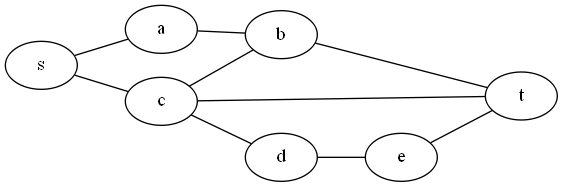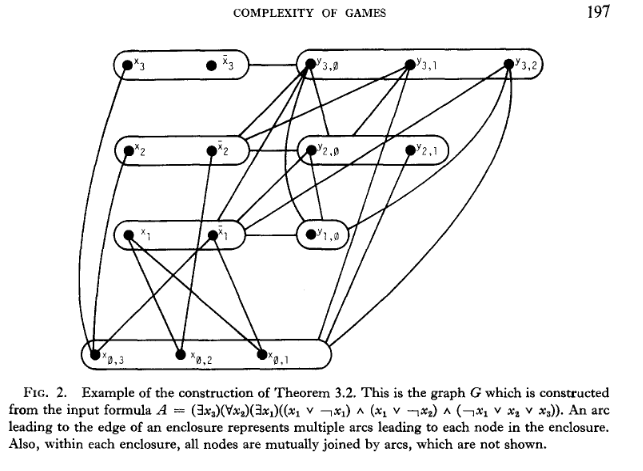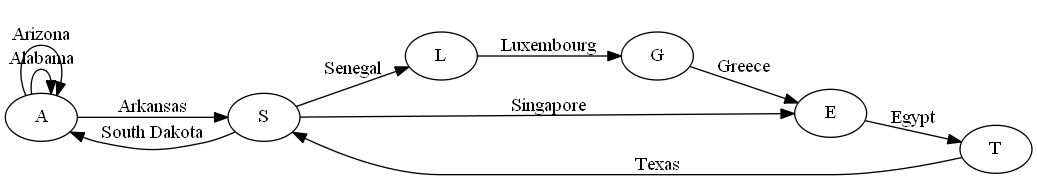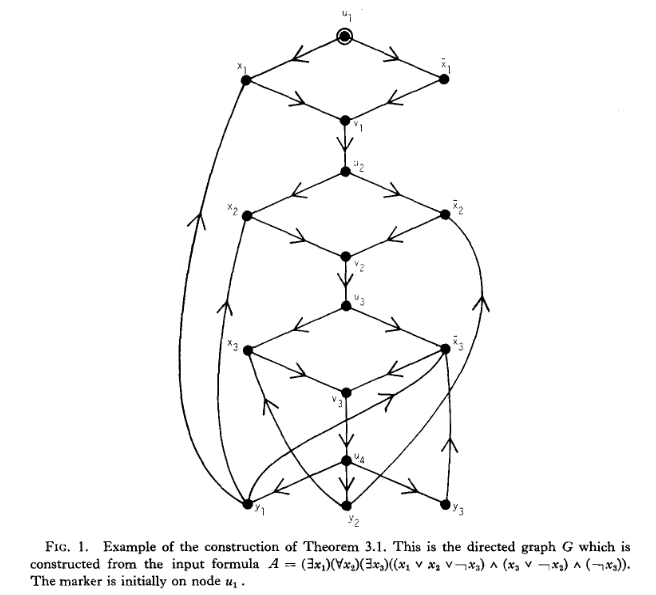# Monthly Archives: March 2019

## Generalized Kayles

I hadn’t heard of this game before encountering this problem.  It sort of feels a lot like Nim to me.

The problem: Generalized Kayles.  This is problem GP3 in the appendix.

The description: Given a graph G=(V,E). Players take turns removing vertices and all vertices adjacent to it from the graph.  The first player to have no vertices left loses.

Example: Here’s a graph:Suppose player 1 chooses vertex c.  Then we also remove s,b, d, and t.  All that is left are vertices a and e.  Whichever vertex player 2 chooses, player 1 can choose the other one, and win.

Reduction: This one is again by Schaefer, and again uses the same problem which is either Sequential Truth Assignment or QBF, depending on how you look at it.

Just like last time, we’re given a formula: (∃ x1) (∀ x2) (∃ x3) … (∃ xn) (A1 ∧ A2 ∧ … Am), where each Ai is a disjunction of literals.  We’ll further assume n is odd (so the last quantifier is ∃).  The graph is built as follows:

• Each clause k in the formula gets a vertex x0,k.  These vertices are all connected to each other.
• Each variable xi in the formula gets two vertices: xi and ~xi, that have an edge between them. We also get y vertices: yi,j for all j 0 <= j < i
• We add an edge between xi and x0,k if xi appears as a literal in clause k.  Similarly, we add an edge between ~xi and x0,k if ~xi appears as a literal in clause k.
• Each yi,j vertex connects to all xk vertices where k <= i.  Each yi,j vertex also connects to all ya,b vertices where a < i and b < a.

Here’s a picture from the paper of what we get:One main point that comes out of this construction is that players need to take their first n moves playing the x (or ~x) vertices in order.  If you go out of order, the opponent can play a y vertex and remove everything.  If you play a y vertex, the opponent can play an x vertex (or an x0 vertex) and remove everything.

If the original formula was satisfiable, player 1 (who is the ∃ player) starts by choosing either x1 or ~x1.  No matter which of x2 or ~x2 is chosen by the opponent (the ∀ player), player3 will have a way to set x3 to keep the formula satisfiable.  This process continues for all variables.  Once player 1 plays the last variable (xn or ~xn), all vertices have been removed from the graph- most importantly, all of the x0 vertices have been removed, because there is an edge from each one to each variable whose setting would satisfy the clause.  Thus, player 2 has no place to play, and player 1 wins.

If the formula is not satisfiable, then after taking turns choosing xi or ~xi for all i, there is still some x0 vertex in the graph (corresponding to a clause not satisfied by the variable choices made).  Player 2 can select that vertex, removing all x0 vertices from the graph, and will win.

Difficulty: 7.  I can see what the “jobs” of each vertex are: The xi  set the truth values of a variable, the x0 ensure that each clause is satisfied by a variable setting, and the y vertices are there to force players to choose the xi vertices in order.  I don’t think I could have come up with this though.

## Generalized Geography

It’s spring break here- I was expecting to take the week off, but got this post done anyway.  This might mean I skip next week though.

The problem: Generalized Geography.  This is problem GP2 in the appendix.

The description: Given a directed graph G=(V,A) and a starting vertex vo.  Players alternate choosing edges that leave the current vertex (starting with v0.  The next current vertex is the one at the end of the edge leaving from vo.  You can’t choose an edge that is already chosen.  The first player to be unable to choose an edge loses.  Does Player 1 have a forced win?

Example: This is the “geography” game kids play in the car, where you have to think of a place that has as its first letter the last letter of the previous choice.  As a graph problem, vertices correspond to letters, and edges to locations:Note that the vertices can have self-loops, and (at least in the actual game) could have multiple edges between pairs of vertices.  There is no requirement to have any edges leave a vertex either- I remember instituting the “Phoenix rule”  on car trips because nobody could think of a place that started with X.

Anyway, if we start at E, player 1 has a forced win- they have to take “Egypt” to T, and then player 2 has to take “Texas” to S, and if player 1 chooses “Singapore” we’re in vertex E, it’s player 2’s turn, and they have no place left to pick.  (The actual game has many more edges, of course)

Reduction:  Schaefer says he’s building off of the Sequential Truth Assignment problem from last time, but his instance looks more like an instance of QBF. (I think this is a result of his claim that they’re basically the same problem).  So we’re given a formula: (∃ x1) (∀ x2) (∃ x3) … (∃ xn) (A1 ∧ A2 ∧ … Am), where each Ai is a disjunction of literals.  We’ll further assume n is odd (so the last quantifier is ∃)

We then go on to build a graph.  Each positive and negative literal gets a vertex.  Each variable x1 gets 4 vertices in the graph:

• xi corresponding to a positive literal
• ~xi corresponding to a negative literal
• 2 vertices u and v that control the “entrance” and “exit” for the setting of one of the 2 literal values.

We have a vertex for each clause (yi), and an additional vertex un+1 so the last vi has someplace to go.

Each set of these 4 vertices are set up in a diamond, with edges from ui to both xi and ~xi, and then from both of those to vi. vi then connects to ui+1, making a chain of diamonds. The final un+1 connects to each y vertex, and each y vertex connects to the literals that are in its clause.

Here’s the example picture from the paper:Player 1 starts on vertex u1. The player chooses whether to make variable x1 positive or negative by going to that vertex (simulating a “there exists”), and player 2 has no choice but to move on to the v1 vertex, and player 1 has no choice to move to the next u2 (simulating a “for all”). This alternating process continues until we hit vertex un+1.  Since there are an odd number of variables, it is now player 2’s turn.

If the setting of the variables has not satisfied some clause, player 2 can move to the y vertex of that unsatisfied clause.  Then player 1 has to go to one of the 3 literals that appear in that clause.  Since the clause was not satisfied, all of these variables have not been chosen, so the edge from that literal to the v vertex is available for player 2 to pick.  But after that, the vertex from the v vertex to the next u vertex has already been chosen, so player 1 loses.

If the setting of the variables has satisfied every clause, then player 2 has to pick a move to a y vertex that has a literal chosen by a player to satisfy it. When player 1 moves to that vertex, the only edge exiting that vertex has already been chosen, so player 2 loses.

Difficulty: 7. I think this is very slick and elegant, but I don’t see a student getting there without lots of help.

## Protected: Sequential Truth Assignment

This content is password protected. To view it please enter your password below:

## Protected: Generalized Hex

This content is password protected. To view it please enter your password below: